Homework Help Question & Answers

# (8 marks) Problem (2) Liquid Ethanol (C2H5OH) is burned with 100% theoretical air Determine the theoretical...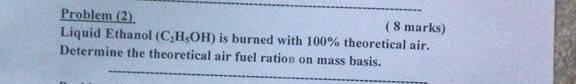(8 marks) Problem (2) Liquid Ethanol (C2H5OH) is burned with 100% theoretical air Determine the theoretical air fuel ration on mass basis.

#### Homework Answers

Answer #1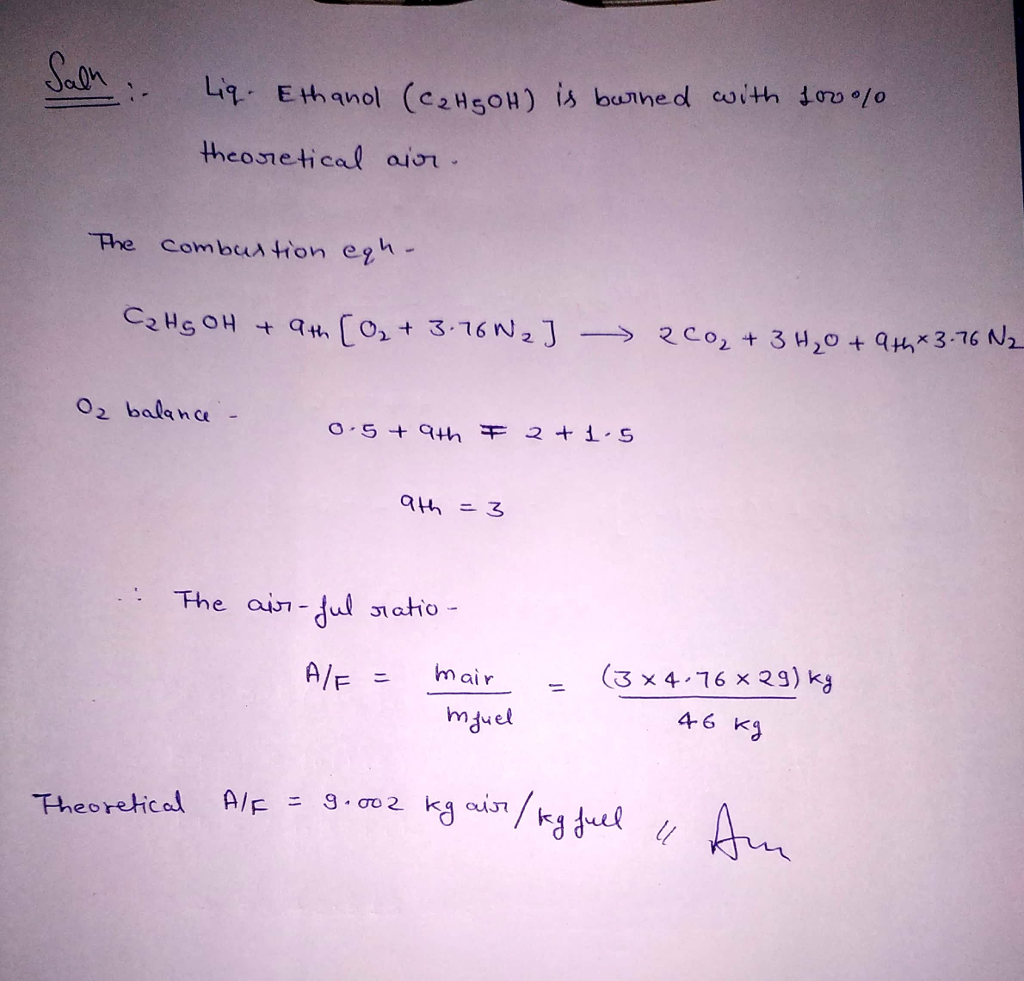Know the answer?
Your Answer:

#### Post as a guest

Your Name:

What's your source?

#### Earn Coin

Coins can be redeemed for fabulous gifts.

Not the answer you're looking for? Ask your own homework help question. Our experts will answer your question WITHIN MINUTES for Free.
Similar Homework Help Questions
• ### (12 marks) Problem (3) Gas Ethanol (C2H5OH) is burned with 100% theoretical air. The fuel enters at 100 KPa, 25 °C and the air enters at 100 KPa, 27 °C. The temperature of the products is 800 k. D...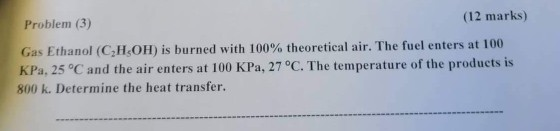(12 marks) Problem (3) Gas Ethanol (C2H5OH) is burned with 100% theoretical air. The fuel enters at 100 KPa, 25 °C and the air enters at 100 KPa, 27 °C. The temperature of the products is 800 k. Determine the heat transfer. (12 marks) Problem (3) Gas Ethanol (C2H5OH) is burned with 100% theoretical air. The fuel enters at 100 KPa, 25 °C and the air enters at 100 KPa, 27 °C. The temperature of the products is 800 k....

• ### (9 marks) Problem (3) Pentane (C5H12) is burned with 200% theoretical air. The air and the fuel e...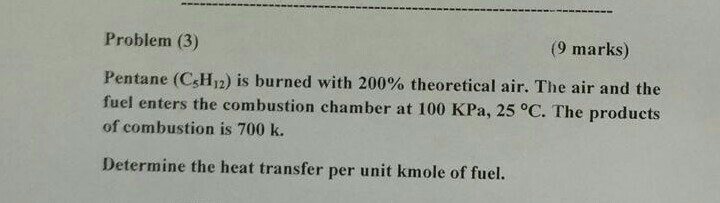(9 marks) Problem (3) Pentane (C5H12) is burned with 200% theoretical air. The air and the fuel enters the combustion chamber at 100 KPa, 25 °C. The products of combustion is 700 k. Determine the heat transfer per unit kmole of fuel. (9 marks) Problem (3) Pentane (C5H12) is burned with 200% theoretical air. The air and the fuel enters the combustion chamber at 100 KPa, 25 °C. The products of combustion is 700 k. Determine the heat transfer per...

• ### (9 marks) Problem (3) Pentane (C5H12) is burned with 200% theoretical air. The air and the fuel e...(9 marks) Problem (3) Pentane (C5H12) is burned with 200% theoretical air. The air and the fuel enters the combustion chamber at 100 KPa, 25 °C. The products of combustion is 700 k. Determine the heat transfer per unit kmole of fuel. (9 marks) Problem (3) Pentane (C5H12) is burned with 200% theoretical air. The air and the fuel enters the combustion chamber at 100 KPa, 25 °C. The products of combustion is 700 k. Determine the heat transfer per...

• ### Problem 4a) An air-breathing gas-turbine jet engine operates at SSSF with complete combustion of 1 kmol/s of liquid ethanol fuel (C2H5OH). The given air-fuel ratio is 33 kg-air/kg-fuel. The constant...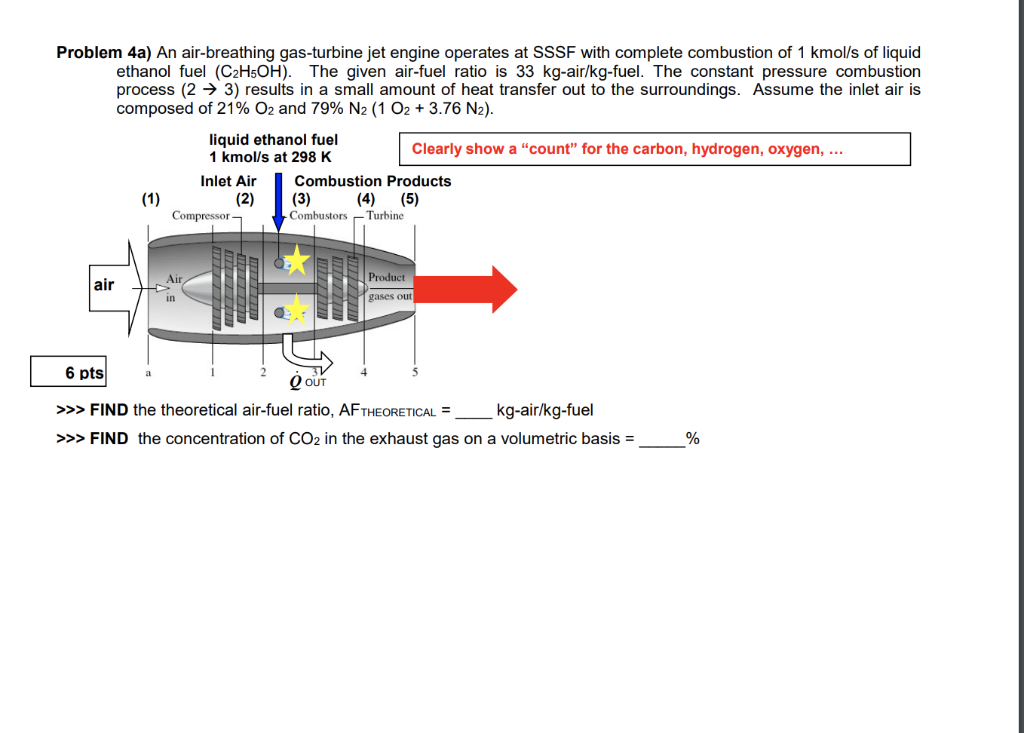Problem 4a) An air-breathing gas-turbine jet engine operates at SSSF with complete combustion of 1 kmol/s of liquid ethanol fuel (C2H5OH). The given air-fuel ratio is 33 kg-air/kg-fuel. The constant pressure combustion process (2 -> 3) results in a small amount of heat transfer out to the surroundings. Assume the inlet air is composed of 21% O2 and 79% N2(1 O2+ 3.76 N2) liquid ethanol fuel 1 kmol/s at 298 K Clearly show a "count" for the carbon, hydrogen, oxygen,......

• ### 5 liters of methanol (CH3OH) and 4 liters of ethanol (C2H5OH) are mixed and burned with...

5 liters of methanol (CH3OH) and 4 liters of ethanol (C2H5OH) are mixed and burned with 4000 gram moles of air. Not all of the hydrocarbons are burned in the furnace, and both CO and CO2 are formed. (a) The fuels went through both complete and incomplete burning. List all the equations happening for the combustion reaction. (b) Calculate the theoretical oxygen that should be supplied to the furnace. (c) Calculate the percent excess air supplied to the furnace. (d)...

• ### Problem (5) A Solid Sulfur (S) is burned with 100% theoretical air. The sulfur enters the combustion chamber at P=100 KPa, 25 oC. The air enters at P=100 KPa, T-770C. The temperature of the produc...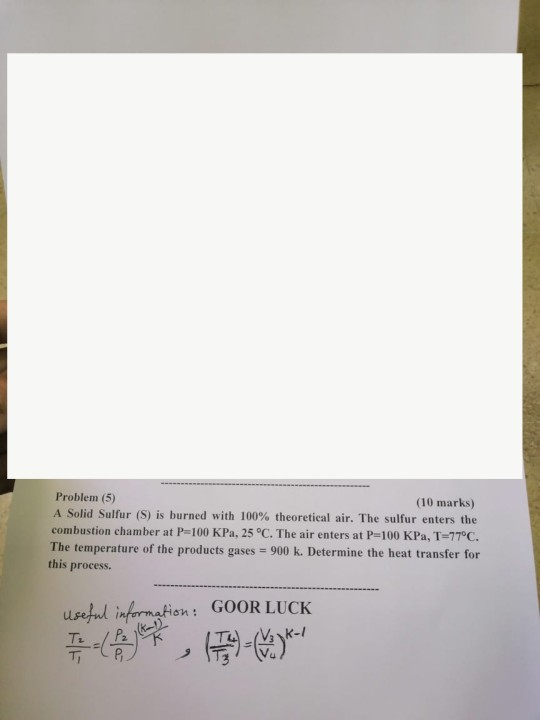Problem (5) A Solid Sulfur (S) is burned with 100% theoretical air. The sulfur enters the combustion chamber at P=100 KPa, 25 oC. The air enters at P=100 KPa, T-770C. The temperature of the products gases - 900 k. Determine the heat transfer for this process. (10 marks) GOOR LUCK useful informaki 4 Problem (5) A Solid Sulfur (S) is burned with 100% theoretical air. The sulfur enters the combustion chamber at P=100 KPa, 25 oC. The air enters at...

• ### (9 marks) Proben tane (C4H10) is burned with 200% theoretical air. The r- Butane (CH of...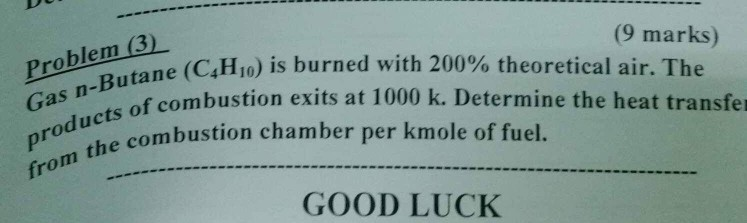(9 marks) Proben tane (C4H10) is burned with 200% theoretical air. The r- Butane (CH of combustion exits at 1000 k. Determine the heat transfe prod the combustion chamber per kmole of fuel. GOOD LUCK

• ### (a) Consider methane is burned in an internal combustion engine using 200% theoretical air. Locate the...

(a) Consider methane is burned in an internal combustion engine using 200% theoretical air. Locate the following unknown parameters: I. Air/fuel ratio on mass basis II. Composition of products III. Dew point temperature of the products

• ### Answer all the questions please Problem 1 (20 pts) (No Partial Credits) i. Butane, CaHio, is burned with air at an air fuel ratio of 20. Determine the percentage of excess ii. Benzene gas, CsHi, i...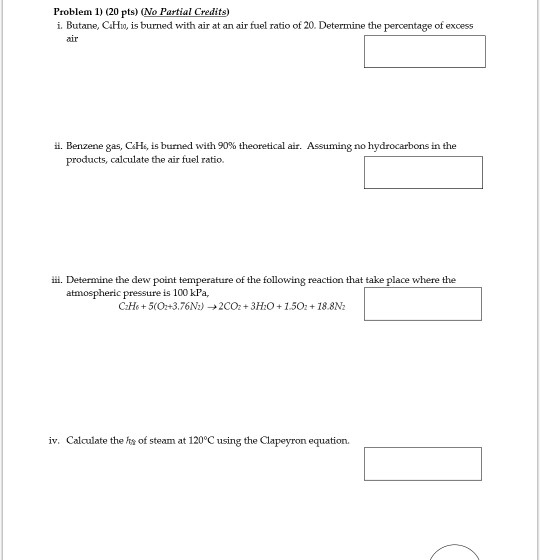Answer all the questions please Problem 1 (20 pts) (No Partial Credits) i. Butane, CaHio, is burned with air at an air fuel ratio of 20. Determine the percentage of excess ii. Benzene gas, CsHi, is burned with 90% theoretical air. Assuming no hydrocarbons in the products, calculate the air fuel ratio. ill. Determine the dew point temperature of the following reaction that take place where the atmospheric pressure is 100 kPa, iv. Calculate the rs of steam at 120°C...

• ### A 12.8 g sample of ethanol (C2H5OH) is burned in a bomb calorimeter with a heat capacity of 5.65 kJ/�C. Using the inform...

A 12.8 g sample of ethanol (C2H5OH) is burned in a bomb calorimeter with a heat capacity of 5.65 kJ/�C. Using the information below, determine the final temperature of the calorimeter if the initial temperature is 25.0�C. The molar mass of ethanol is 46.07 g/mol.

Free Homework App

Scan Your Homework
to Get Instant Free Answers
Need Online Homework Help?

Get Answers For Free
Most questions answered within 3 hours.